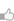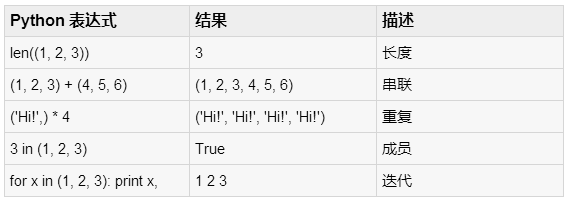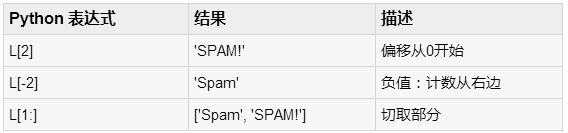求知 文章 文库 Lib 视频 iProcess 课程 认证 咨询 工具 讲座 Modeler Code要资料追随技术信仰 随时听讲座 每天看新闻

 Python教程 Python快速入门 Python概述 Python环境安装 Python基本语法 Python变量类型 Python基本运算符 Python决策 Python循环 Python数据类型 Python字符串 Python列表 Python元组 Python字典 Python日期时间Date/Time Python函数 Python模块 Python文件I/O Python异常处理 高级教程 Python 3开发网络爬虫(一) Python 3开发网络爬虫(二) Python 3(三): 伪装浏览器 Python 3(四): 登录 Python面向对象 Python正则表达式 Python CGI编程
Python元组
 388 次浏览43次捐助

 `tup1 = ('physics', 'chemistry', 1997, 2000);tup2 = (1, 2, 3, 4, 5 );tup3 = "a", "b", "c", "d";`

 `tup1 = ();`

 `tup1 = (50,);`

 `#!/usr/bin/python` tup1 = ('physics', 'chemistry', 1997, 2000); tup2 = (1, 2, 3, 4, 5, 6, 7 ); print "tup1: ", tup1 print "tup2[1:5]: ", tup2[1:5]

 `tup1: physicstup2[1:5]: [2, 3, 4, 5]`

 `#!/usr/bin/python` tup1 = (12, 34.56); tup2 = ('abc', 'xyz'); # Following action is not valid for tuples # tup1 = 100; # So let's create a new tuple as follows tup3 = tup1 + tup2; print tup3;

 `(12, 34.56, 'abc', 'xyz')`

 `#!/usr/bin/python` tup = ('physics', 'chemistry', 1997, 2000, hema); print tup; del tup; print "After deleting tup : " print tup;

 `('physics', 'chemistry', 1997, 2000)After deleting tup :Traceback (most recent call last): File "test.py", line 9, in print tup;NameError: name 'tup' is not defined``L = ('spam', 'Spam', 'SPAM!')``#!/usr/bin/python` print 'abc', -4.24e93, 18+6.6j, 'xyz'; x, y = 1, 2; print "Value of x , y : ", x,y;

 `abc -4.24e+93 (18+6.6j) xyzValue of x , y : 1 2`

Python中包括以下元组的功能：

 SN 方法及描述 1 cmp(tuple1, tuple2) 添加obj对象到列表 2 len(tuple) 计算返回obj出现在列表的次数 3 max(tuple) 附加序列seq内容到列表 4 min(tuple) 返回列表中出现obj的最小索引 5 tuple(seq) 插入obj对象在列表偏移索引位置
 您可以捐助，支持我们的公益事业。 1元 10元 50元 认证码：必填
 388 次浏览43次捐助